﻿ Estimation of E-Field inside Muscle Tissue at MICS and ISM Frequencies Using Analytic and Numerical Methods

### Estimation of E-Field inside Muscle Tissue at MICS and ISM Frequencies Using Analytic and Numerical ...

Yessar E. Mohammed, Ali G. SaberOPEN ACCESSPEER-REVIEWED

## Estimation of E-Field inside Muscle Tissue at MICS and ISM Frequencies Using Analytic and Numerical Methods

Yessar E. Mohammed1, Ali G. Saber2,1Department of Electrical Engineering, University of Mosul, College of Engineering, Mosul, Iraq

2Department Electrical Engineering, University of Mosul, College of Engineering, Mosul, Iraq

### Abstract

This paper presents studying for analytic and numerical methods which are used for evaluating the magnitude of electric field inside human tissues for different depths and using the most important frequencies in medical application fields, then showing the difference range between the two methods. The first method considers the human tissue as one-dimensional layered, the layers in this method modeled with non uniform transmission line. The second method is using numerical simulation CST Microwave Studio which considers the human tissues as 3D-dimensional layered. This paper also investigates the penetration for the magnitude of electric field into the phantom model of human tissues, especially into muscle tissue using different frequencies and how the electric field behaves inside the tissue when the penetration depth greater than the thickness of the tissue, as well as when the penetration depth smaller than the thickness of the tissue.

### At a glance: Figures

123
Prev Next

• Mohammed, Yessar E., and Ali G. Saber. "Estimation of E-Field inside Muscle Tissue at MICS and ISM Frequencies Using Analytic and Numerical Methods." Journal of Biomedical Engineering and Technology 2.3 (2014): 29-33.
• Mohammed, Y. E. , & Saber, A. G. (2014). Estimation of E-Field inside Muscle Tissue at MICS and ISM Frequencies Using Analytic and Numerical Methods. Journal of Biomedical Engineering and Technology, 2(3), 29-33.
• Mohammed, Yessar E., and Ali G. Saber. "Estimation of E-Field inside Muscle Tissue at MICS and ISM Frequencies Using Analytic and Numerical Methods." Journal of Biomedical Engineering and Technology 2, no. 3 (2014): 29-33.

 Import into BibTeX Import into EndNote Import into RefMan Import into RefWorks

### 1. Introduction

Over the last decades several techniques have been developed to analyze interactions between incident electromagnetic wave and human tissue, generally the human tissue divided into multilayer [1, 2]. The electric properties of human tissue vary with frequency changing [3, 4, 5]. There are many bands used in the medical fields. In this research study focused on MICS Medical Implant Communication System (402MHz) and ISM (Industrial Scientific and Medical) bands which commonly used in the diagnostic and therapeutic applications. ISM bands were originally reserved internationally for the use of RF electromagnetic fields for industrial, scientific and medical purposes other than communications, ISM includes the frequencies (434MHz, 915MHz,2.45MHz, 5.8GHz) [9, 10, 11, 12, 13]. The main purpose of the recent researches is estimating the specific absorption rate (SAR) in the tissues [14, 15]. For evaluating SAR at certain depth, we need to find E-field at certain frequency and apply equation (1) .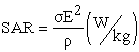(1)

where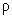represents the conductivity of tissue (S/m),the density of the tissue (kg/m3) and E the electric field(V/m). There are many applications for evaluating the E-field. The most three important applications are .

•  Therapeutic applications, such as hyperthermia by focusing EM-field at certain point.

•  Diagnostic applications, EM-fields at microwave frequencies can provide a convenient approach to detect and monitor physiological.

•  Studying the effects of Electromagnetic that penetrates the tissue such as using mobile and micro-wave frequency.

There are several methods for evaluating the electric field in biological tissue. The simple method considers the tissue as homogeneous medium, then by analyzing the transmission characteristics of multilayer structure using non uniform transmission line method [6, 7, 8], where the characteristic impedances and transmission coefficients of each layers are calculated, then applying the non uniform transmission line method to calculate the parameter of ABCD network [6, 7, 8]. Other method is using CST software for evaluating the electric field inside human tissue. The CST software applies FIT technique (Finite Integration Technique). This paper gives interesting for evaluating the magnitude of E-field inside muscle tissue, at different points, and at different frequencies with using two methods. The main reason of choosing muscle tissue is the wide thickness of this tissue [1, 2]. Thus, the study will be more clarity. The methods which presented in this paper can be used to evaluate the magnitude of E-field inside another tissue.

### 2. Characteristics of Human Tissues

According to previous research, the electrical properties of human tissue are changing with frequency. There is a database available for most parameters of tissues [3, 4]. Table 1, includes information for several tissues and at four frequencies . The penetration depth can be obtained from data base [3, 4] or by applying equation(2) .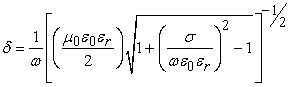(2)

Where: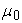free space electromagnetic permeability,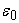free space permittivity,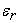relatave permittivity of the tissue,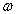wave pulsation, and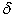the penetration depth.

#### Table 1. Electric Properties of Human Tissue at Different Frequencies 

Figure 1 shows the penetration depth of tissues versus frequency. To penetrate any tissue the penetration depth must be greater than thickness of that tissue.

Figure 1. The relation between frequency range(100MHz-10GHz) and penetration depth for the different tissues by applying equation (2)

The electric field strength decreases according to the equation (3) .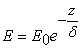(3)

Where: E electric field at certain point, E0 reference electric field, z distance from E0. Inside tissue the E-field will be combining of the wave arrived and the reflected wave from next tissue as in equation (4) .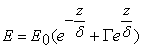(4)

Where Γ represents the reflection coefficient of next tissue, there are more than one reflection accrues inside each tissue, therefore, the matter will be more complicated.

### 3. Analytic Calculation and Numerical Simulation

3.1. First Method

This method considers the human tissues as non uniform transmission lines. The characteristic impedance and transmission coefficient of each tissue can be calculated from equation(5)(6) respectively [6, 7, 8].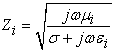(5)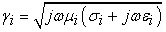(6)

Where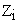and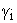are characteristic impedance and transmission coefficient of each layer respectively.

For applying this method we will consider the layers of human as one-dimensional layers as shown in Fig. 2: where Db thickness of bone (20mm), Dm thickness of muscle (20mm), Df thickness of fat (4mm), Ds thickness of skin (2mm), and Dfs free space distance between source and surface of skin layer (10mm), then calculating the parameter of ABCD network using the network parameter matrix of non uniform transmission line in equation (7) [6, 7, 8].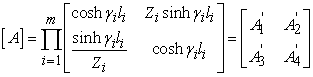(7)

Where m represents number of tissues,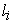the thickness of each tissue. The magnitude of E-field and the impedance of tissue (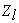) at distance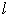to the next tissue can be calculated using equation(8)(9) respectively [6, 7, 8]. Where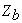free space impedance,impedance of bone,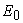incident signal perpendicular to skin, and calculating by equation (9) [6, 7, 8].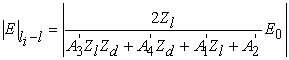(8)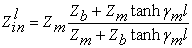(9)

Where: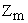impedance of muscle tissue,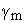transmission coefficient of muscle tissue, and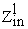input impedance of part of muscle at distance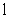to bone tissue.

3.2. Second Method

This method uses CST Microwave Studio which applies Finite Integration Technique. The body phantom designed with dimensions 40mm × 40mm. The structure of phantom model consists of four layers with the same thickness that used in first method. Figure 3 includes the structures of the body phantom that used in this paper.

### 4. Results and Comparison

To compare the results which obtained from the two methods, the tissue parameters, and dimensions should be the same in two methods. The depth into muscle tissue where chosen for comparison at 4, 8, 10, 12, 14 and 18mm. The magnitude of E-field at these points has been calculated for different frequency with normal incident E-field 10V/m to phantom model. For plotting the results we should take more points, therefore, the magnitude of E-field will calculate at each 0.25mm into muscle tissue. When using plane wave with frequency 402MHz, 915MHz and 2.45GHz, the penetration depth of each tissue which used greater than it's thickness, therefore, the signal will penetrate the tissue and reflect from next tissue, causing fluctuation at different point, then forming standing wave. The results are illustrating in Figure 4-a, Figure 4-b, Figure 5-a, Figure 5-b, Figure 6-a and Figure 6-b. The comparison of two methods at different depths and different frequencies illustrated in Table 2, Table 3, Table 4, and Table 5.

#### Table 4. Comparison between two method at 2.45GHz

Figure 4-a. Distribution of magnitude of E-field inside muscle tissue using first method at 402MHz; 4-b. Distribution of magnitude of E-field inside muscle tissue using second method at 402MHz
Figure 5-a. Distribution of magnitude of E-field inside muscle tissue using first method at 915MHz; 5-b. Distribution of magnitude of E-field inside muscle tissue using second method at 915MHz
Figure 6-a. Distribution of magnitude of E-field inside muscle tissue using first method at 2.45GHz; 6-b. Distribution of magnitude of E-field inside muscle tissue using second method at 2.45GHz

When using plane wave of 5.8GHz, the penetration depth according to equation (2) is 7.5413mm. The thickness of muscle tissue that used in this paper 20mm, δ < thickness, therefore, the reflected signal from next tissue will be very small causing exponential fading of signal especially for region from the beginning of the muscle tissue to a depth of 12.45mm, because the signal will behave according to equation (3) with some modification. In this region the transmitted signal much greater than reflected signal, this phenomenon can be observed in Figure 7-a and Figure 7-b. The matter will be different for region from 12.4587 to 20mm, because the signal will behave according to equation (4) with some modification, wherein the reflected signals will be present and must be taken in calculation. This phenomenon causes fluctuation in E-field for region from 12.45mm to 20mm of muscle tissue.

#### Table 5. Comparison between two method at 5.8Hz

Figure 7-a. Distribution of magnitude of E-field inside muscle tissue using first method at 5.8GHz and equation(3); 7-b. Distribution of magnitude of E-field inside muscle tissue using second method at 5.8GHz

### 5. Discussion and Conclusions

A comparison of the two methods gives same results approximately. The difference between results is ranging from 10-3 to 10-2 approximately, this difference can be neglected when using large values of electric field. Therefore, first method is suitable for calculating magnitude of electric field at any point inside muscle or other tissue. Another conclusion is when penetration depth greater than thickness of tissue, the signal will reflect and combine with transmitted signal, therefore, the signal will fluctuate and form standing wave at different points. When penetration depth of muscle smaller than it's thickness, the reflected signal from next tissue will be very small, this causes exponential fading for the signal to certain depth, then the penetration depth will be greater than thickness. The signal will fluctuate at this depth, because appearance of reflected signals.

### References

  N. Vidal and J. M. L´opez, “Changes in Electromagnetic Field Absorption in the Presence of Subcutaneous Implanted Devices: Minimizing Increases in Absorption,” IEEE Trans. Electromag. Compat., Vol. 52, No. 3, August. 2010.In article  G. Varotto and E. M. Staderini, “A 2D Simple Attenuation Model for EM Waves in Human Tissues: Comparison with a FDTD 3D Simu-lator for UWB Medical Radar,” IEEE International Conference on Ultra-Wide Band., Vol. 3, 2008.In article  Federal Communications Commission. Tissue dielectric properties. FCC, Washington, DC. (2008). [Online]. Available: http://www.fcc.gov/fcc-bin/dielec.sh.In article  N. Carrara. Dielectric properties of body tissues. IFAC, Institute for applied physics, Italy. (2007). [Online]. Available:http://niremf.ifac.cnr.it/tissprop/.In article  S. Gabriel, R. U. Lau and C. Gabriel, “The Dielectric Properties of Biological Tissues:ІІ. Measurement in The Frequency Range 10Hz to 20GHz,” Phys. Med. Biol., Vol. 41, pp. 2251-2269, 1996.In article  C. A. Balanis, “Modern Antenna Handbook,” John Wiley & Sons, Inc., 2008.In article CrossRef  S. Dan, G. Yougang and S. Yuanmao, “Determination of Shielding Effectiveness of Multilayer Shield By Making Use of Transmission Line Theory,” 7th IEEE International Symposium on Electromagnetic Compatibility and Electromagnetic Ecology., Petersburg, Russia, pp. 95-97, 2007.In article  D. Shi, Y. Gao, and X. Du, “Study of Human Body Transmission Characteristic as Nonuniform Medium,” IEEE URSI General Assembly and Scientific Symposium., Istanbul, Turkey, pp. 1-4, 2011.In article  Sharing Between the Meteorological Aids Service and Medical ImplantIn article  Communication Systems (MICS) Operating in the Mobile Service in the Frequency Band 401-406 MHz, ITU-R SA.1346, Int. Telecommu-nications Union, 1998.In article  FCC Rules and Regulations, Subpart E and I, Part 95, Federal Comm-unications Commission, Nov. 2002.In article  Relating to the Use of Short Range Devices (SRD), ERC Recomm-endation 70-03, European Radiocommunications Committee, Apr. 2002.In article  Radiocommunications Agency, “UK radio interface requirement 2030 short range devices,” ver. 1.2, Oct. 2002.In article  J. Ung and T. Karacolak, “A Wideband Implantable Antenna for Continuous Health Monitoring in the MedRadio and ISM Band,” IEEE Antennas and Wireless Propagation Letters., Vol. 11, pp. 1642-1645, 2012.In article CrossRef  K. S. Sultan, H. H. Abdullah, E. A. Abdallah, and E. A. Hashish, “ Low-SAR, Miniaturized Printed Antenna for Mobile,ISM, and WLAN Services,” IEEE Antennas and Wireless Propagation Letters., Vol. 12, pp. 1106-1109, 2013.In article CrossRef  S. W. Park, K, Wake, S. Watanable, “ Calculation Errors of the Electric Field Inducedin a Human Body Under Quasi-Static Approximation Conditions,” IEEE Transactions on Microwave Theory and Techniques., Vol. 61, No. 5, pp. 2153-2160, 2013.In article CrossRef  F. S. Barnes and B. Greenebaum, “Bioengineering and Biophysical Aspects of Electromagnetic Fields,” Taylor and Francis Group, LLC., Boca, Raton, London, New York, 2006.In article  G. Kang, O. P. Gandhi, “ Effect of Dielectric Properties on the Peak 1- and 10-g SAR for 802.11 a/b/g Frequencies 2.45 and 5.15 to 5.85 GHz,” IEEE Trans. Electromag. Compat., Vol. 46, No. 2, May. 2010.In article  C. C. Johnson and A. W. Guy, “Nonionizing Electromagnetic Wave Effects in Biological Materials and Systems,” Proceedings of IEEE., Vol. 60, No. 6, pp. 692-718, June. 1972.In article